HomeAtomic SpectroscopyDetermination of Trace Elements following EN 13805 Using ICP-OES

# Determination of Trace Elements following EN 13805 Using ICP-OES

## Introduction

This is a method for the determination of iron, magnesium, potassium, sodium and calcium in foodstuffs. The sample is mineralized through pressurized digestion with nitric acid. In the resulting digestion solution, iron, magnesium, potassium, sodium and calcium are quantified by inductively coupled plasma optical emission spectrometry (ICP-OES).

### Reagents

(only use recognized analytical grades, unless otherwise specified. The concentration of iron, magnesium, potassium, sodium and calcium in the reagents and water used shall be low enough not to affect the results of the determination)

Water, LiChrosolv® solvent (1.15333)

Suprapur® Nitric acid 65% (1.00441)

Multi-element stock solution-Certipur® ICP multi-element standard solution IV (1.11355.0100)

ρ(Fe) = 1,000 mg/L

ρ(Mg) = 1,000 mg/L

ρ(K) = 1,000 mg/L

ρ(Na) = 1,000 mg/L

ρ(Ca) = 1,000 mg/L

### Multi-element Standard and Calibration Solutions

The standard and calibration solutions are prepared from the stock solution by dilution in glass volumetric flasks. For calibration, prepare at least five (5) calibration solutions of different concentrations. The acid concentration shall correspond to the concentration in the measurement solution. The preparation of the solutions below is given as an example.

Calibration solutions of ρ(Fe, Mg, K, Na, Ca) = 0.5, 1.0, 2.5, 5.0, and 10.0 mg/L for ICP-OES.

Fill five 100 mL volumetric flasks with 10-20 mL of water, add 10 mL of nitric acid and mix. Cool the solutions to ambient temperature, and pipette exactly 0.05, 0.1, 0.25, 0.5, 1.0 mL of multi-element stock solution for the respective calibration solutions of mass concentrations 0.5 mg/L,

1.0 mg/L, 2.5 mg/L, 5.0 mg/L, 10.0 mg/L into the five different 100 mL volumetric flasks.
Mix the solutions and dilute to volume with water.

The calibration solutions described here shall be understood as examples. The concentrations prepared shall be in the linear range of the measuring device. The acid concentration of the calibration solutions shall be matched to the acid concentration in the sample solution.

### Blank Solution

The blank solution contains water, nitric acid in amounts that correspond to the concentrations in the measurement solution, for example 10 mL of nitric acid in 100 mL of water.

### Apparatus

• Microwave Reaction System
• ICP- OES (Axial viewing).

### Digestion of the Sample

Mineralize the sample in pressurized digestion in accordance with EN 13805:2014. The digestion requirements are based on the specifications of the instrument manufacturer, the reactivity of the sample, the maximum pressure stability of the digestion vessel and the attainable temperature. Precisely weigh 0.8-0.9 g of sample in digestion vessel and mix it 5 mL nitric acid and 1 mL water. The digestion solution that results from the pressurized digestion according to the norm can be used directly or can be diluted for the subsequent quantification of iron, magnesium, potassium, sodium and calcium.

### Inductively Coupled Plasma Optical Emission Spectrometry (ICP–OES)

Start the instrument and let it stabilize, then optimize it according to the manufacturer’s specifications and begin measurements. Use the blank solution to zero the instrument. Use the calibration solutions and measure the emission of the element to be determined.

### Evaluations

Relevant analytical lines and the limits of quantification (LOQ) using ICP–OES.

Calibration Data – Calcium (Ca)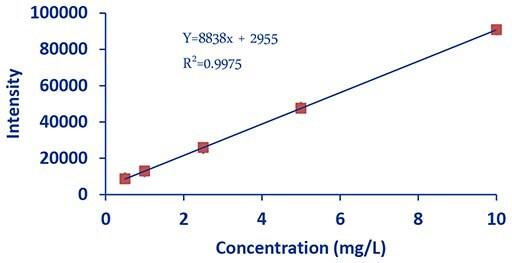Figure 1.DCE Calcium

Calibration Data – Sodium (Na)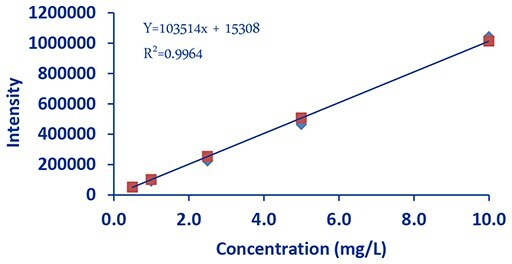Figure 2.DCE Sodium

Calibration Data – Magnesium (Mg)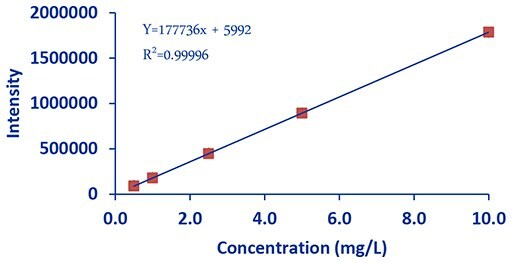Figure 3.DCE Magnesium

Calibration Data – Iron (Fe)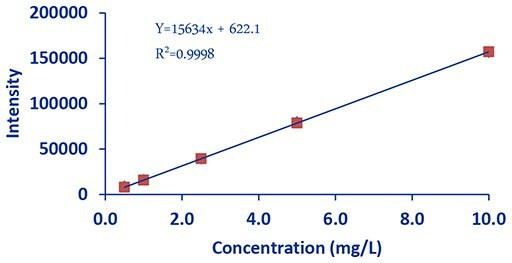Figure 4.DCE Iron

Calibration Data –Potassium (K)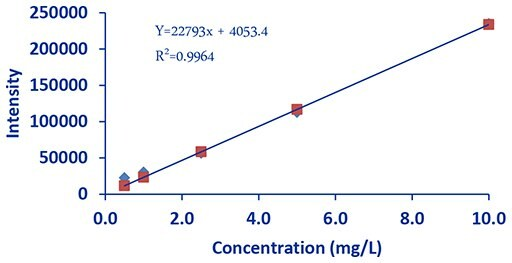Figure 5.DCE Potassium

## Results

The table below present mean values of Iron (Fe), Magnesium (Mg), Calcium (Ca), Sodium (Na) and Potassium (K) found in the three different type of samples.

Materials